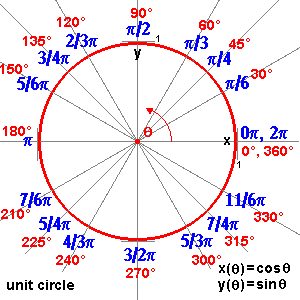Home    |    Teacher    |    Parents    |    Glossary    |    About UsEmail this page to a friendResources· Cool Tools · Formulas & Tables · References · Test Preparation · Study Tips · Wonders of MathSearchCircles (Math | Geometry | Circles)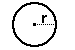a circle
Definition: A circle is the locus of all points equidistant from a central point.

Definitions Related to Circles

 arc: a curved line that is part of the circumference of a circle chord: a line segment within a circle that touches 2 points on the circle. circumference: the distance around the circle. diameter: the longest distance from one end of a circle to the other. origin: the center of the circle pi (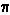): A number, 3.141592..., equal to (the circumference) / (the diameter) of any circle. radius: distance from center of circle to any point on it. sector: is like a slice of pie (a circle wedge). tangent of circle: a line perpendicular to the radius that touches ONLY one point on the circle.
Diameter = 2 x radius of circle

Circumference of Circle = PI x diameter = 2 PI x radius
where PI == 3.141592...

Area of Circle:
area = PI r2Length of a Circular Arc: (with central angle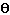)
if the angleis in degrees, then length =x (PI/180) x r
if the angleis in radians, then length = r xArea of Circle Sector: (with central angle)
if the angleis in degrees, then area = (/360)x PI r2
if the angleis in radians, then area = ((/(2PI))x PI r2

Equation of Circle: (Cartesian coordinates)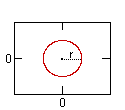for a circle with center (j, k) and radius (r):
(x-j)^2 + (y-k)^2 = r^2

Equation of Circle: (polar coordinates)
for a circle with center (0, 0):   r() = radius

for a circle with center with polar coordinates: (c,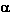) and radius a:
r2 - 2cr cos(-) + c2 = a2

Equation of a Circle: (parametric coordinates)
for a circle with origin (j, k) and radius r:
x(t) = r cos(t) + j       y(t) = r sin(t) + k